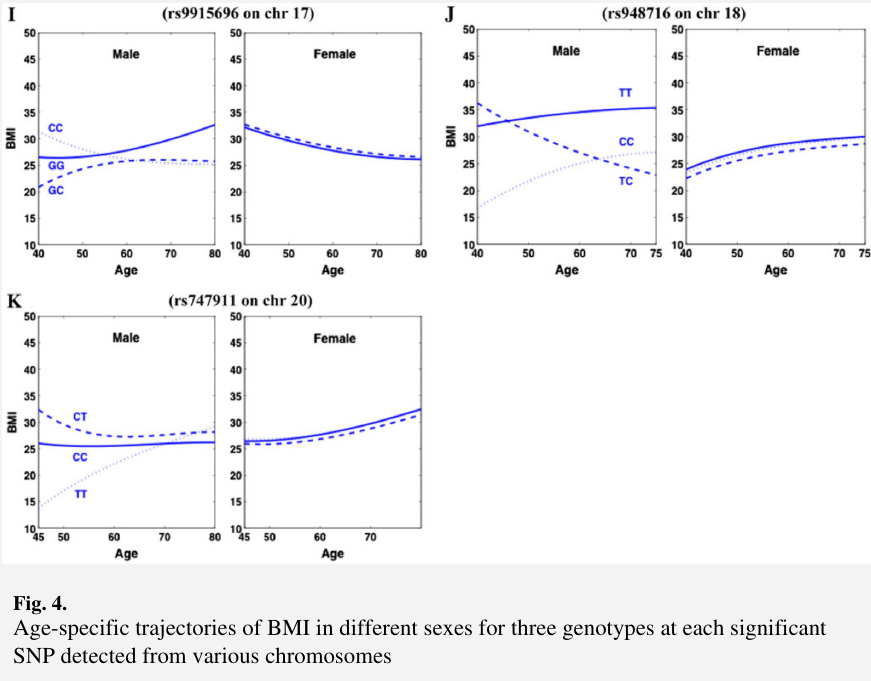# fGWAS: Dynamic Model for GWAS

##### Posted on Jun 11, 2023 (Update: Jul 27, 2023)
Tags: GWAS, Time-varying

In the fGWAS of clinical data sets, longitudinal traits are measured at irregular and possibly subject-specific time points.

$y_i(t_{i\tau}) = \sum_{j=1}^3\xi_i\mu_j(t_{i\tau}) + \beta^T(t_{i\tau})x_i + e_i(t_{i\tau}) + \varepsilon_i(t_{i\tau})$

where

• $\mu_j(t_{i\tau})$: mean value for genotype $j$ at time $t_{i\tau}$## another GWAS

$y_i(t_{i\ell}) = \mu(t_{i\ell}) + \alpha(t_{i\ell})^TX_i + a(t_{i\ell})^T\xi_i + d(t_{i\ell})^T\zeta_i + e_i(t_{i\ell})$

where

• $\xi_{ij} = 1 I(AA) + 0 I(Aa) - 1 I(aa)$
• $\zeta_{ij} = I(Aa) + 0 I(AA) + 0 I(aa)$

Published in categories Note